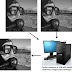Results for Wavelet transform

Multi-Focus Image Fusion Matlab Code Using Wavelet Transform (SWT)

January 22, 2022
Image Fusion Matlab Code |  Image Fusion | Types of  Image Fusion |  Single-Sensor Image Fusion | Multi-Sensor Image Fusion | Multi-View ...
Multi-Focus Image Fusion Matlab Code Using Wavelet Transform (SWT)Reviewed by IPR on January 22, 2022 Rating: 5

MATLAB implementation of Multi-focus image fusion using wavelet transform

April 18, 2020
MATLAB implementation of Multi-focus image fusion using wavelet transform 1. Image fusion is one of the most researched area in the ...
MATLAB implementation of Multi-focus image fusion using wavelet transformReviewed by IPR on April 18, 2020 Rating: 5

Implementation of DWT/ SWT on image using MATLAB

April 18, 2020
Implementation of Discrete wavelet transform (DWT) and  Stationary wavelet transform (SWT) on an image using MATLAB 1. In Matlab, for ...
Implementation of DWT/ SWT on image using MATLABReviewed by IPR on April 18, 2020 Rating: 5